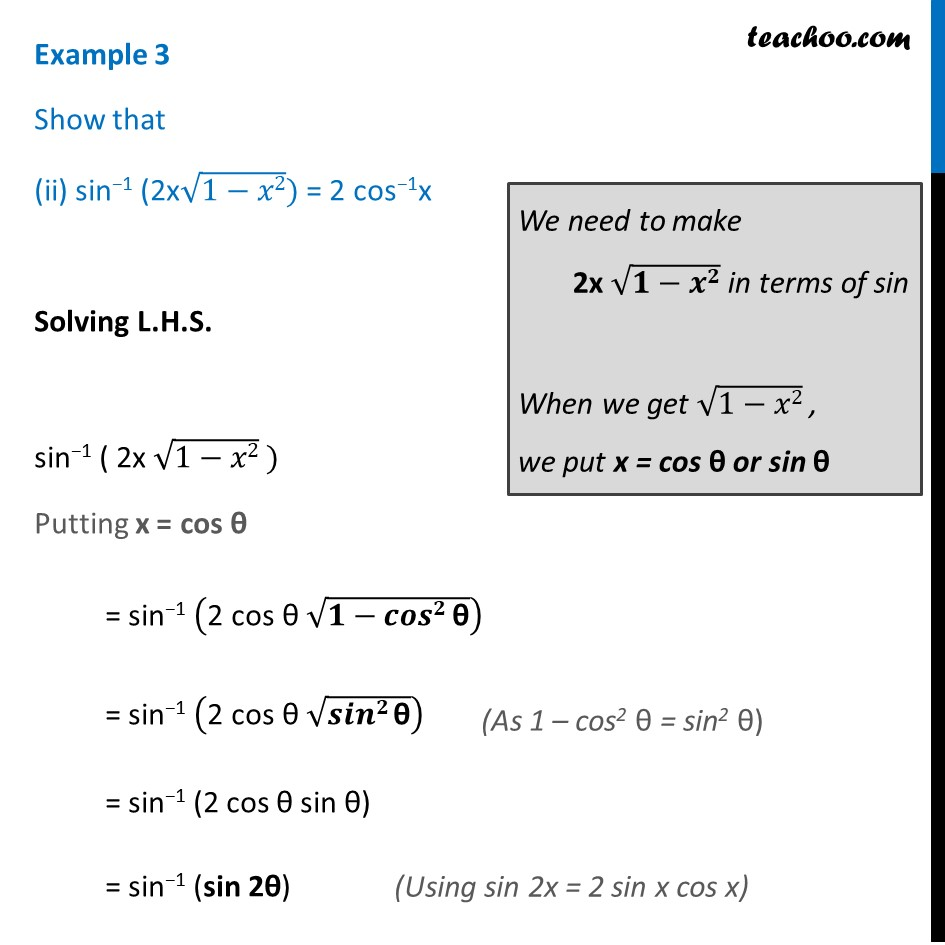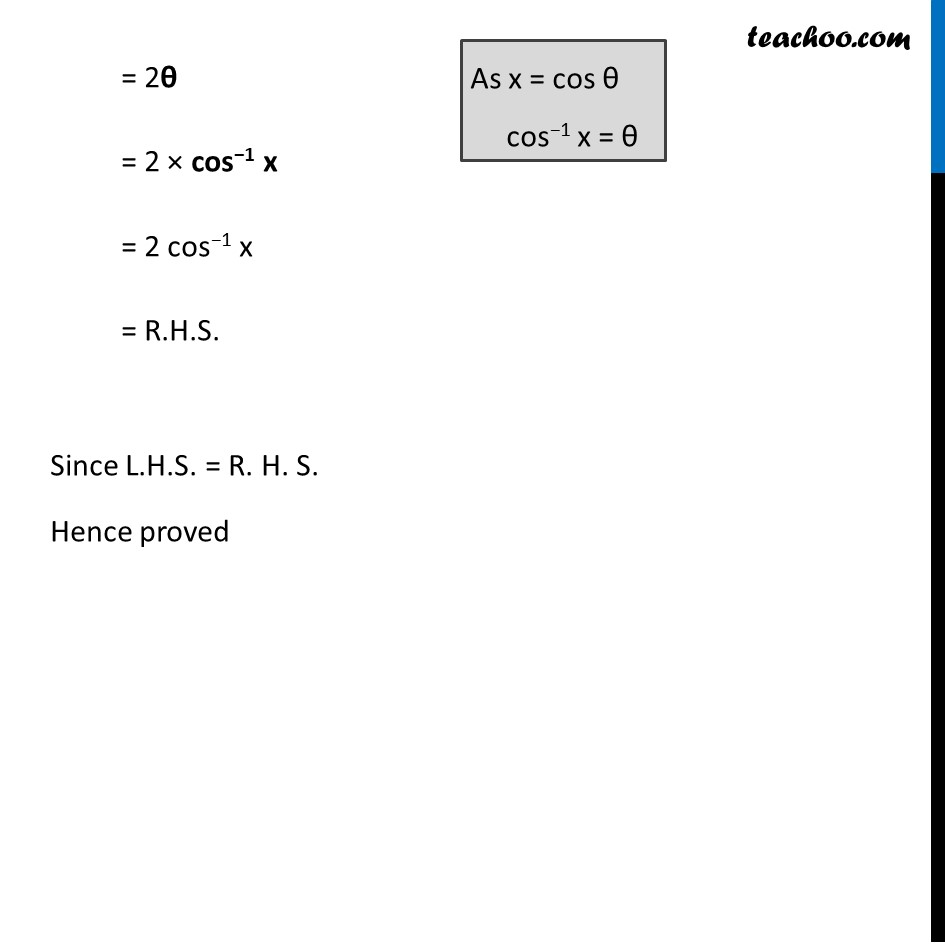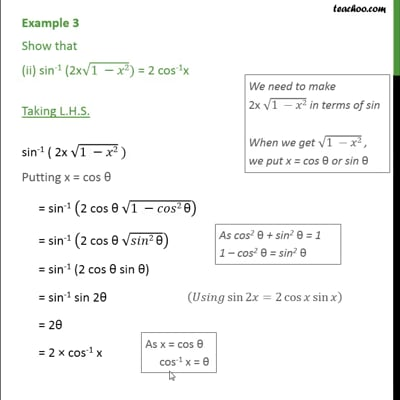Examples

Chapter 2 Class 12 Inverse Trigonometric Functions
Serial order wiseThis video is only available for Teachoo black users

Maths Crash Course - Live lectures + all videos + Real time Doubt solving!

### Transcript

Example 3 Show that (ii) sin−1 (2x√(1−𝑥2)) = 2 cos−1x Solving L.H.S. sin−1 ( 2x √(1−𝑥2) ) Putting x = cos θ = sin−1 ("2 cos θ " √(𝟏−𝒄𝒐𝒔𝟐" θ" )) = sin−1 ("2 cos θ " √(𝒔𝒊𝒏𝟐" θ" )) = sin−1 (2 cos θ sin θ) = sin−1 (sin 2θ) We need to make 2x √(𝟏−𝒙𝟐) in terms of sin When we get √(1−𝑥2) , we put x = cos θ or sin θ = 2θ = 2 × cos−1 x = 2 cos−1 x = R.H.S. Since L.H.S. = R. H. S. Hence proved As x = cos θ cos−1 x = θ